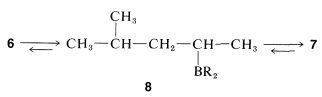# 13.8: Introducing Functionality

$$\newcommand{\vecs}{\overset { \rightharpoonup} {\mathbf{#1}} }$$ $$\newcommand{\vecd}{\overset{-\!-\!\rightharpoonup}{\vphantom{a}\smash {#1}}}$$$$\newcommand{\id}{\mathrm{id}}$$ $$\newcommand{\Span}{\mathrm{span}}$$ $$\newcommand{\kernel}{\mathrm{null}\,}$$ $$\newcommand{\range}{\mathrm{range}\,}$$ $$\newcommand{\RealPart}{\mathrm{Re}}$$ $$\newcommand{\ImaginaryPart}{\mathrm{Im}}$$ $$\newcommand{\Argument}{\mathrm{Arg}}$$ $$\newcommand{\norm}{\| #1 \|}$$ $$\newcommand{\inner}{\langle #1, #2 \rangle}$$ $$\newcommand{\Span}{\mathrm{span}}$$ $$\newcommand{\id}{\mathrm{id}}$$ $$\newcommand{\Span}{\mathrm{span}}$$ $$\newcommand{\kernel}{\mathrm{null}\,}$$ $$\newcommand{\range}{\mathrm{range}\,}$$ $$\newcommand{\RealPart}{\mathrm{Re}}$$ $$\newcommand{\ImaginaryPart}{\mathrm{Im}}$$ $$\newcommand{\Argument}{\mathrm{Arg}}$$ $$\newcommand{\norm}{\| #1 \|}$$ $$\newcommand{\inner}{\langle #1, #2 \rangle}$$ $$\newcommand{\Span}{\mathrm{span}}$$$$\newcommand{\AA}{\unicode[.8,0]{x212B}}$$

As we have seen in the previous section, it may be easy to construct the carbon skeleton of the target compound of a synthesis, but with a reactive functional group at the wrong carbon. Therefore it is important also to have practice at shifting reactive entry points around to achieve the final desired product. We shall illustrate this form of molecular chess with reactions from previous post. A typical problem may be to devise syntheses for achieving the following conversions:If you proceed as in the previous section, you will see that the starting material and products have the same number of carbons and the same general bonding arrangement of those carbons. Getting the functionality to the right carbons is now the problem. If you review the reactions discussed up to now, you will find that the only good way of getting a reactive group at the end of a chain starting with a reactive group in the middle of the chain is borane isomerization (Section 11-6C). The borane, $$6$$, can be obtained from the starting material, $$5$$, by hydroboration (Section 11-6) and, on heating, $$6$$ will be converted to $$7$$:Production of 4-methyl-1-pentanol from the substituted alkylborane, $$7$$, can be achieved by oxidation (Section 11-6D):The three steps - hydroboration, isomerization, and oxidation - thus constitute a reasonable synthesis of the first desired compound.

The second desired product is a little more tricky because the isomerization of $$6$$ to $$7$$ cannot be stopped at the alkylborane, $$8$$:The best procedure to get the desired product is to generate the 1-alkene from the borane with 1-decene (Section 11-6C) and then add hydrogen bromide by a polar mechanism (Section 10-4). Incursion of radical-chain addition must be avoided because it would give 1-bromo-4-methylpentane (Section 10-7):

A very brief summary of the transformations that we have studied so far, which do not change the carbon skeleton, is given in Table 13-5 along with appropriate section references. In using this table, it is necessary to check the specific sections to be sure the reaction is applicable to the conversion that you wish to achieve and to determine the proper conditions for the reaction.

Table 13-5: Summary of Useful Synthetic Transformations